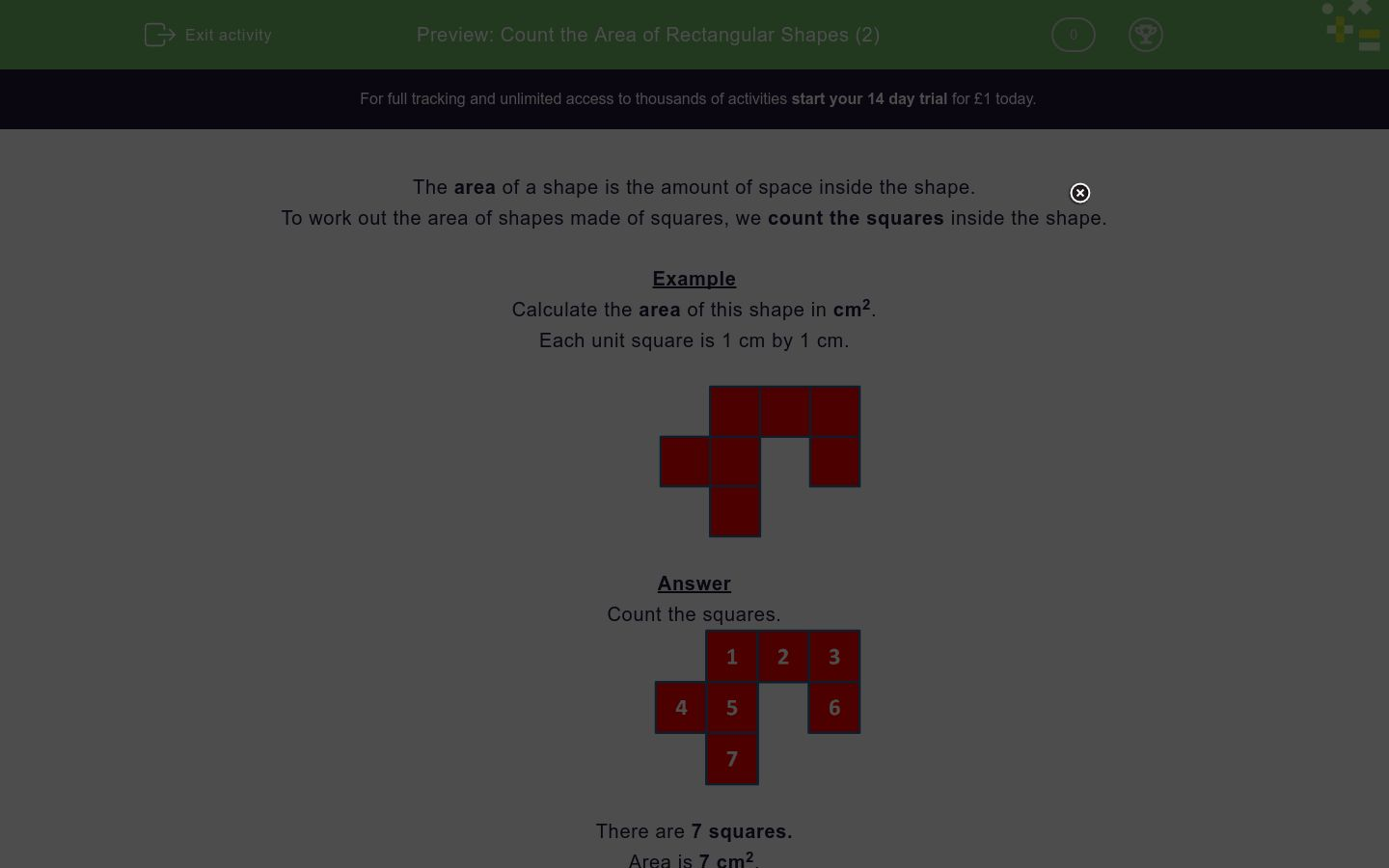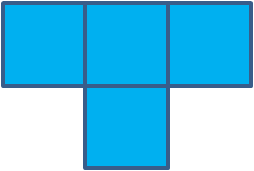# Count the Area of Rectangular Shapes (2)

In this worksheet, students must calculate the area of rectilinear shapes made of cm or m squares by counting the squares.Key stage:  KS 2

Curriculum topic:   Measurement

Curriculum subtopic:   Calculate Areas

Difficulty level:### QUESTION 1 of 10

The area of a shape is the amount of space inside the shape.

To work out the area of shapes made of squares, we count the squares inside the shape.

Example

Calculate the area of this shape in cm2.

Each unit square is 1 cm by 1 cm.Count the squares.There are 7 squares.

Area is 7 cm2.

We read this as seven centimetres squared.

Remember

If the lengths are in metres, the area will be in m2.

Want to understand this further and learn how this links to other topics in maths?
Why not watch this video?

Calculate the area of this shape in cm2.

Each unit square is 1 cm by 1 cm.Just write the number.

Calculate the area of this shape in m2.

Each unit square is 1 m by 1 m.Just write the number.

Calculate the area of this shape in cm2.

Each unit square is 1 cm by 1 cm.Just write the number.

Calculate the area of this shape in m2.

Each unit square is 1 m by 1 m.Just write the number.

Calculate the area of this shape in cm2.

Each unit square is 1 cm by 1 cm.Just write the number.

Calculate the area of this shape in m2.

Each unit square is 1 m by 1 m.Just write the number.

Calculate the area of this shape in cm2.

Each unit square is 1 cm by 1 cm.Just write the number.

Calculate the area of this shape in m2.

Each unit square is 1 m by 1 m.Just write the number.

Calculate the area of this shape in cm2.

Each unit square is 1 cm by 1 cm.Just write the number.

Calculate the area of this shape in m2.

Each unit square is 1 m by 1 m.Just write the number.

• Question 1

Calculate the area of this shape in cm2.

Each unit square is 1 cm by 1 cm.Just write the number.

4
EDDIE SAYS
There are 4 centimetre squares.
• Question 2

Calculate the area of this shape in m2.

Each unit square is 1 m by 1 m.Just write the number.

4
EDDIE SAYS
There are 4 metre squares.
• Question 3

Calculate the area of this shape in cm2.

Each unit square is 1 cm by 1 cm.Just write the number.

5
EDDIE SAYS
There are 5 centimetre squares.
• Question 4

Calculate the area of this shape in m2.

Each unit square is 1 m by 1 m.Just write the number.

5
EDDIE SAYS
There are 5 metre squares.
• Question 5

Calculate the area of this shape in cm2.

Each unit square is 1 cm by 1 cm.Just write the number.

5
EDDIE SAYS
There are 5 centimetre squares.
• Question 6

Calculate the area of this shape in m2.

Each unit square is 1 m by 1 m.Just write the number.

6
EDDIE SAYS
There are 6 metre squares.
• Question 7

Calculate the area of this shape in cm2.

Each unit square is 1 cm by 1 cm.Just write the number.

8
EDDIE SAYS
There are 8 centimetre squares.
• Question 8

Calculate the area of this shape in m2.

Each unit square is 1 m by 1 m.Just write the number.

9
EDDIE SAYS
There are 9 metre squares.
• Question 9

Calculate the area of this shape in cm2.

Each unit square is 1 cm by 1 cm.Just write the number.

8
EDDIE SAYS
There are 8 centimetre squares.
• Question 10

Calculate the area of this shape in m2.

Each unit square is 1 m by 1 m.Just write the number.

8
EDDIE SAYS
There are 8 metre squares.
---- OR ----

Sign up for a £1 trial so you can track and measure your child's progress on this activity.

### What is EdPlace?

We're your National Curriculum aligned online education content provider helping each child succeed in English, maths and science from year 1 to GCSE. With an EdPlace account you’ll be able to track and measure progress, helping each child achieve their best. We build confidence and attainment by personalising each child’s learning at a level that suits them.

Get started